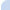Home Produkte Katalog FAQ Über uns Kontakte
 Rus | Eng | De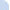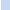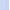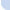# Optics Definitions

## Absorbance

log 10 (Io / Ie) Example: 20% transmittance is log 10 (100 / 20) = 0.7 absorbance.

## Absorptance

Intensity absorbed beam / Intensity incident beam = Complement of transmittance (Io - Ie) / Io

## Bulk Modulus (K)

This relates to changes in total volume of material.

Changes in volume / Original volume (dV/Vo)

## Bulk stress

Change in pressure / Area (dP/A)

## Chamfers

Chamfers are usually required both to protect the work from chipping during polishing and also in use. Chamfers are normally 45-deg and measured along the flat of the chamfer. Protective chamfers are usually referred to as 'break-edge' and are typically 0.25mm. They are regarded as uncritical and often removed by the polishing process. Unless otherwise stated, normal chamfering is manufactured as 0.5mm.

## Cosmetic Finish

Cosmetic Finish is often a subjective matter and despite more recent sophisticated methods of classification is still commonly specified by the traditional method of Scratch & Dig which tries to classify the surface in terms of the maximum length of small scratches left after polishing. Under this classification S/D 80/60 would be regarded as quite poor and appropriate to simple spectroscopic windows, while S/D 20/10 is a quite exacting standard suitable for low scattering laser applications. Inevitably assessment of S/D tends to err on the conservative side; 60/40 being a normal routine finish.

## Design of Pressure Windows

The calculation of minimum thickness of a window to withstand a pressure gradient should be approached with caution. The published figures for apparent elastic limit, flexural strength, or rupture modulus may be used but it should be realised that these three terms relate to different methods of test. Further, the published data should not be considered absolute as theses samples from which the original data was obtained may not be representative of all samples of crystal. A conservative safety factor should always be applied to the minimum calculated thickness therefore.

For a circular window, avoiding plastic deformation, the minimum design thickness is indicated by:

tmin = K x [(p x D2) / S ]½

For an unclamped window K = 1.06
For a clamped window K = 0.866

D is the unsupported diameter
S is the apparent elastic limit
p is the pressure differential

Calculate thickness of the windows in our Excel sheet.

## Elastic Coefficients

Elastic coefficients, otherwise known as Elastic Stiffness Constants are the constants of proportionality between the components of stress and strain. They are therefore related to the elastic moduli.

These coefficients are designated by the term Chk where h and k are integers between 1 and 6.

For cubic crystals the coefficients are C11, C12, C44
For tetragonal crystals, five, C11, C12, C13, C33, C44
For hexagonal crystals, six, C11, C12, C13, C14, C33, C44

The three moduli relate to these coefficients as follows:

Youngs modulus = E = (C11 + 2C12) (C11 - C12) * / (C11 + C12)

Bulk modulus = K = (C11 + 2C12) / 3

Shear modulus = G = C44

* This is for the particular case of a rod cut parallel to the edges of the unit cell. For the general case it is...

1 / E = (C11 + C12) + (C11 - C12 - 2C44) / (C11 + 2C12) * (C11 + C12)

D = (nx2.ny2 + nx2 .nz2 + ny2.nz2)

Where nx, ny and nz are the cosines of the angles between the direction of the longitudinal lengthening of the rod and the cubic cell edges.

## Elastic Moduli

The deformation of a material can be quantified by expressing it in terms of the elastic modulus. If deformations are small, the material will retain memory and return to original shape when the pressure or stress causing the deformation has been removed.

Robert Hooke, a founder member of the Royal Society, discovered that the deformation of a material was proportional to the load or force applied and this was formulated as Hooke's Law.

An elastic modulus is defined as Stress/Strain.

## Flatness

Flatness is specified in terms of the wavelength of light, eg Lambda/2 at 633nm, which represents the degree of power, with sometimes a form-error addition, eg Lambda/5 at 633nm, which defines the allowed irregularity of the fringe pattern. Polishing flat to 1 fringe normally assumes sodium light or HeNe laser and corresponds to a deviation of about 0.25 microns. The wavelength should always be stated.
TIP: 1 Fringe = Lambda/2

## Hardness Scales

Hardness is a well known physical parameter, but many different methods have been derived for the measurement and classification of materials on a hardness scale. The Knoop scale is the most commonly used, the others being; Moh, Vickers, Rockwell and Brinell.

The experimental procedure for the derivation of a value on the Knoop scale is to use a pyramidal diamond point which is pressed into the material in question with a known force. The indentation made by the point is then measured and the Knoop number calculated from this measurement. The test has been designed for use on a surface that has not been work-hardened in the lattice direction in which the hardness value is being measured.

The value of the Knoop number varies slightly with the indenter load as well as with the temperature. A material which is soft, i.e. potassium bromide, would have a Knoop number of 4, whereas a hard material like sapphire has a Knoop number of 2000 and the Knoop number for diamond is 7000.

The values on the Moh scale are arrived at by measuring the relative hardness of materials by observing which materials are able to scratch other materials. The Moh scale, which is not linear, is limited by the softest material Talc (Moh=1) and the hardest material Diamond (Moh=10).

The Vickers scale is determined by pressing a pyramidal indenter into the material in question and dividing the indenter load (in kg) by the pyramidal area of the indenter (in mm2).

Rockwell and Brinell hardness are not often quoted. The Rockwell figures for materials are relative to a specific measuring instrument and the Brinell hardness is analogous to the Vickers scale except that a spherical indenter is used.

## Lenses

Lenses involve variations on the specifications above. "Flatness" translates into a matter of a match of the radius of curvature to a reference standard known as a "test-plate" while "Parallelism" can still be measured as a "run-out" but is more commonly specified as a centration error.

## Metrological Lasers

HeNe Laser = 0.6328µm CO2 Laser = 10.6µm

## Normal Atmospheric Pressure

(1) pounds/sq inch 14.7psi
(2) inches of mercury 29.9213"
(3) mm of mercury 760mm
(4) millibars 1013.240mBar
Now measured in SI units:
(5) Pascals 101.324kPa

(2) and (3) are straightforward measures of a column of mercury supported by 1 atmosphere: 1" = 25.4mm 1mm = 0.03937"

(4) and (5) are actual measures of pressure (force/area) and take account of the density of mercury (13.595gm/cc at 0-degC) and the acceleration due to gravity (980.665 cm/sec2) when converting from a mercury barometer reference.

Pressure at 1 atm = 0.76m x 13595 kgm.m-3 x 9.80665 m . S-1
= 101324 Pa (N/m2)(m-1.kgm.s-1)
101.324kPa

## Optic Axis

All crystalline materials, except those which belong to the cubic structure class, are anisotropic to a greater or lesser degree. Anisotropic (non- isotropic) crystals possess different physical properties in different directions of measurement.

In anisotropic crystals there is a single direction which is an axis of symmetry with respect to both the crystal form and the arrangement of atoms in the lattice. This axis of symmetry is called the Optic Axis. If any physical property of the material, thermal conductivity for example, is measured in different directions, it is found to have a value particular to that direction. If measured along any line perpendicular to the optic axis the value of the physical property will change as the direction of measurement changes, reaching a maximum or minimum along the optic axis.

Birefringence (double refraction) in uniaxial crystals disappears when light is made to travel along the optic axis, as there is no separation of the E-ray and the O-ray. This birefringent property of uniaxial crystals gives rise to phenomena of polarisation when the E and O rays are polarised in perpendicular planes.

## Parallelism

Parallelism of a window can be specified in terms of angle, usually as minutes of arc. Sometimes it is in terms of thickness variation or "run-out". With conventional polishing techniques where no particular control of this parameter is involved, parallelism of less than 10 to 20 arcminutes can be expected. Where double sided techniques are involved, parallelism obtained are usually not measurable by normal methods. In some cases, to avoid multiple reflections between the faces, a small wedge angle may actually be specified.

## Poisson Ratio (µ)

The Poisson ratio relates to the fractional change in cross sectional dimension and the fractional change in length of material under stress.

µ = Lateral contraction/Original diam = Transverse strain Longitudinal extension/Original length = Longitudinal strain

Where a rod is stretched the cross-sectional area decreases as length increases and this is usually accompanied by a change in volume.

µ = - R/Ro Where Ro = Original diameter L/Lo Lo = Original length

The negative sign is used to give Poisson's ratio a positive value. It can be shown that for a material which deforms with no change in volume, Poisson's ratio is equal to 0.5. For a decrease in volume, the ratio is greater than 0.5 and for an increase it is less than 0.5.

## Reflection Loss

When light is incident upon a dielectric interface, part of it is reflected. The reflection loss is a ration usually specified as a percentage of the original intensity. The loss is a function of the refractive index (n) of the material, the state of polarisation and the angle of incidence of the light.

The reflection loss, from one surface, of un-polarised light, normally incident upon a surface is given by:-

Reflection Loss = (n - 1)2 / (n + 1)2

For transparent materials, reflections from the second surface must be considered. Taking into account the possible multiple internal reflections, the internal transmittance of a parallel plate is given by:-

Transmittance = 2n / (n2 + 1)

Reflection loss decreases as the angle of incidence increases. Normal incidence being the condition for minimum reflection loss.

## Reststrahlen

The Reststrahlen (residual rays) effect, occurs notably in the 6-micron - 300-micron range. For many inorganic materials used in optical applications, the refractive index remains constant and the absorption coefficient is zero or very small, over the majority of its transmission range. When Reststrahlen materials are illuminated at wavelengths that excite the atomic, or crystal lattice structure, a number of quite dramatic resonance effects are noticed. As the Reststrahlen maximum frequency is approached the refractive index (n) undergoes a rapid change, the extinction coefficient (k) rises rapidly and the Fresnel reflection coefficient (R) may become quite high. Therefore, at Reststrahlen maximum frequency of the particular material in question, the reflectance of that material rises sharply.

R = (n - 1)2 + k2 / (n + 1)2 + k2

In the 6 to 300-micron region where the Reststrahlen effects seem to be most prominent, there are not many satisfactory filters. Hence the Reststrahlen effect is often used for the extraction of a narrow spectral region from a spectral continuum.

In practical applications some properties of Reststrahlen materials are quite significant. Particularly the fact that the maximum reflectance increases as the temperature is reduced and when used as thin films as coatings on mirrors and lenses, they exhibit interference maxima and minima, on the short wavelength side of their reflectance peaks.

## Shear or Rigidity Modulus (G)

This relates to the tangential force per unit area and is again expresses as the ratio of stress to strain.

## Shear strain

Angle of shear = dx / y

## Shear stress

Tangential Force / Area (F/A)

## Specific Heat

The specific heat of a material is defined as the ratio between the specific heat capacity of that material to the specific heat capacity of water.

The specific heat capacity of a material is defined as the quantity of heat required to raise a unit mass of the material through one degree Kelvin, with no phase change occurring.

Specific heat is a function of temperature but in the temperature ranges usually encountered by optical materials it can be regarded as effectively constant.

## Strain

Change in length / Original length (dL/Lo)

## Stress

Tensile Force / Area (F/A)

## Transmissivity

Internal Transmittance per unit thickness

## Transmittance

Intensity of exit beam / Intensity of incident beam (le / lo)
Transmittance is usually expressed as a percentage.

## Wave Number

1 / wavelength (cm) = 10000 / wavelength (micron)

## Young Modulus (E)

This relates to tensile or compressive force per unit area and can be defined as the ratio of stress to strain, where the stress is perpendicular to the direction of the applied force and the strain is the fractional change in length.Tel: +7 (812) 363 12 40 Email: technologies@alkor.net